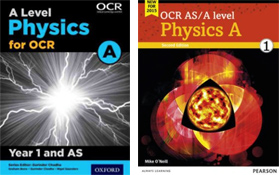Translate

An award winning sixth-form college in the heart of Central London offering a wide range of GCSE and A-Level courses

The quality of the curriculum, teaching and learners' achievements is excellent
ISI INSPECTION REPORT - APRIL 2023

T (+44) 20 7409 7273
Albemarle Independent College, 18 Dunraven Street, Mayfair, London W1K 7FE# PHYSICS A (OCR)

AS Course Outline

What is Physics?As you probably know from GCSE, Physics is the science of the physical world. There are few completely new topics at AS-level that you won't have come across. AS level physics is simply GCSE work on mechanics, electricity and waves in more detail. Most GCSE Higher Level calculation topics are simply repeated, e.g. acceleration and kinetic/potential energy, voltage-current-resistance, while others e.g. heat, energy, electromagnetism and astronomy have calculations introduced at A-level, but are only descriptive at GCSE. Maths is involved in A-level Physics, and it is highly recommended that Maths is taken in tandem with Physics (you will even repeat the same Mechanics material in both Maths and Physics!). However, you don't need A Level Maths to be successful in Physics, you just need to be comfortable with most of the higher-tier GCSE maths, e.g. formulae (using and re-arranging them), graphs e.g. y=mx+c, Pythagoras, sin, cos and tan percentages and standard form.

AS-level Physics involves mechanics; motion, forces, momentum, kinetic/potential energy, and stretching solids, Hooke's Law. We study electricity, both circuits and how current is explained by electrons, then waves (e.g. light and sound waves). Other topics include refraction, diffraction, interference and sound waves in musical instruments. We study the wave and particle photon nature of light in quantum physics.

In the second year of the course, moving from AS to A Level, you will study further mechanics topics e.g. circular motion and oscillations, heat energy, the kinetic molecule theory of gases, astronomy-planet orbits, star life cycles, the Big Bang theory of the Universe, electromagnetism (motors, generators, transformers), radioactivity, particle physics and medical physics (e.g. how X-Ray machines work). There is no “coursework” or practical examinations to worry about. You will do experiments throughout the course and answer exam questions about these in the exams. There will be two exam papers to do in May/June. There will be multiple-choice and structured questions with a mix of calculations and descriptive work. You may need to write a paragraph to explain something but no essays. The whole AS syllabus, modules 1-4 will be examined in both papers. You get a comprehensive formula sheet in the exams.

Students will face questions based on:

• Calculation from formula
• Describe, explain or define.
• Describe familiar experiments
• Analyse experiment data e.g. plotting a graph

What Skills Will Students Develop by Studying Physics?

• Numerical skills
• Mathematical skills-graph gradients, rearranging equations (GCSE algebra)
• Problem-solving skills
• Practical/experimental skills

 PHYSICS (OCR) Syllabus A H156 Summary of Content at AS Course Content Module 1: Development of Practical Skills in Physics Physics is a practical subject. The development and acquisition of practical skills is fundamental. Students are introduced to experimental design, analysis and evaluation techniques. Skills learned in this part of the course are vital for those who wish to study science and engineering courses at university. Module 2: Foundations in Physics The aim of this module is to introduce important conventions and ideas that permeate the fabric of physics. Understanding of physical quantities, S.I. units, scalars and vectors helps physicists to effectively communicate their ideas within the scientific community Module 3: Forces and Motion This develops the mathematical foundation to much of what is learned at GCSE, including momentum, moments, work done and efficiency. Students are also introduced to studies involving Young's modulus, which is a key link to civil and mechanical engineering. Practicals completed in this part of the course include methods to determine g on Earth, determining the terminal velocity of materials, and investigating the properties of plastics. Module 4: Electrons, waves and photons Building upon GCSE electricity and waves, students examine more complicated electric circuits and gain a greater understanding the electrical systems of every day objects, as well as furthering their knowledge in wave behaviour, refraction and diffraction. Students gain their first exposure to quantum physics and study the phenomenon of wave-particle duality. Practicals completed in this part of the course include investigating potential divider circuits, determine the wavelength of light using a diffraction grating, and determining the speed of sound in air.

Course Structure
Students will have 5 hours of contact time per week, covering the course content, practical work, and exam practice.

Core Textbook
OCR Year 1 and AS Level ISBN 9780198352174 Oxford University Press